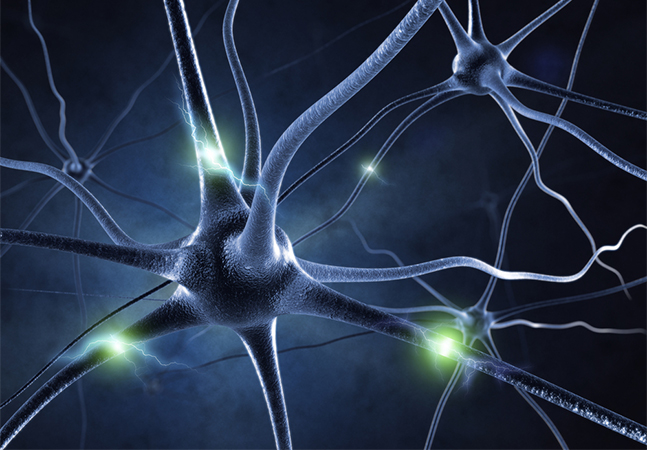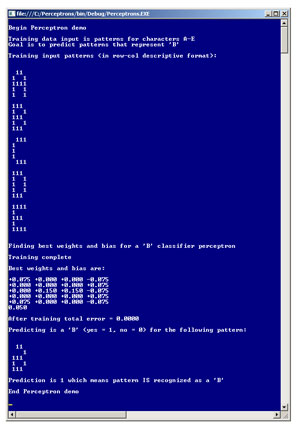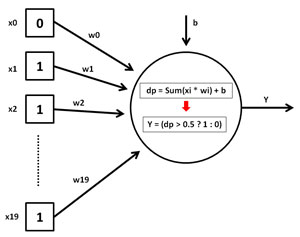#Neural Network Lab

### Modeling Neuron Behavior in C#

James McCaffrey presents one of the basic building blocks of a neural network.A perceptron is code that models the behavior of a single biological neuron. Perceptrons are the predecessors of neural networks. A neural network can be thought of as a collection of connected perceptrons. Learning about perceptrons might be useful to you for at least five reasons, in my opinion.

1. Perceptrons can be used to solve simple but practical pattern-recognition problems.
2. Understanding perceptrons provides you with a good foundation for learning about neural networks, which are very complex.
3. Knowledge of perceptrons is almost universal for anyone who works in the field of machine learning.
4. Several of the techniques used in programming perceptrons can be useful in other problem scenarios.
5. You might simply enjoy learning about software based on the behavior of a biological system.

The best way to see where this article is headed is to look at Figure 1. The image shows a demo console application that creates a perceptron that predicts whether an input pattern of 20 zeros and ones matches those representing a text-graphical representation of a 'B' character. The demo program has five training patterns. Four patterns do not represent a 'B' and one pattern does. The perceptron uses the training data to determine 20 weight values plus a single bias value. These 21 values essentially define the behavior of the perceptron. The perceptron is then presented with an unknown pattern, which, if you look closely, you can see is a 'B' pattern damaged in two bit positions. The perceptron classifies the unknown pattern, and in this case believes the pattern does represent a 'B.'[Click on image for larger view.] Figure 1. The perceptron pattern recognition demo.

In the sections that follow, I'll carefully explain the code that generated the screenshot in Figure 1. I use C# with static methods, but you shouldn't have too much trouble refactoring my code to another language or another object-oriented programming (OOP) style if you wish. This article assumes you have at least intermediate-level programming skill, but does not assume you know anything about perceptrons.

How Perceptrons Work
One way to visualize how perceptrons work is shown in Figure 2. The x0 through x19 represent inputs. In the case of the 'A' pattern shown in Figure 1, there are 20 inputs, four values for each of five rows: 0, 1, 1, 0, . . . 1, 0, 0, 1. The w0 through w19 represent weights that must be determined, one for each input. The 20 weights in Figure 1 are +0.075, +0.000, . . . -0.075. There is a single b (which stands for bias) value, which is a constant that must be determined. The bias value is 0.050.[Click on image for larger view.] Figure 2. How perceptrons work.

The output of a perceptron, Y, is computed in three steps. First, the product of all inputs, x, times their associated weights, w, are summed. Second, the bias is added to the sum. This is labeled dp, which stands for dot product. Third, if the dot product is greater than 0.5, the output Y is 1; otherwise the output is 0. The third step is called the activation function, and the 0.5 value is called the threshold value for the function. An alternative to outputting 0 or 1 is to output -1 or +1.

The perceptron's prediction for the bit pattern for the damaged 'B' pattern is computed as follows:

```dp = (0)(0.075) + (1)(0.00) + (1)(0.00) + (0)(-0.75) + ... + (0)(-0.75) + 0.050
= 0.525 + 0.050
= 0.575

Y  = (dp > 0.5 ? 1: 0)
= 1```

Once a perceptron has values for its weights and bias, computing an output is easy. The main challenge is to determine the best values for the weights and bias. This process is called training the perceptron.

Overall Program Structure
To create the demo perceptron program, I launched Visual Studio 2012 and created a new C# console application project named Perceptrons. The demo program has no significant dependencies, so any version of Visual Studio and the Microsoft .NET Framework should work fine. In the Solution Explorer window, I renamed file Program.cs to the more descriptive PerceptronProgram.cs, and Visual Studio automatically renamed class Program, too. I deleted all template-generated using statements except the one referencing the System namespace.

The overall structure of the program, with a few minor edits, can be seen in Listing 1. The demo program has a Main method and seven helper methods.

Listing 1. The overall perceptron demo program structure.

```using System;
namespace Perceptrons
{
class PerceptronProgram
{
static void Main(string[] args)
{
try
{
Console.WriteLine("\nBegin Perceptron demo\n");
int[][] trainingData = new int[];
trainingData = new int[] { 0, 1, 1, 0, 1, 0, 0, 1, 1, 1,
1, 1, 1, 0, 0, 1, 1, 0, 0, 1,   0 };  // 'A'
trainingData = new int[] { 1, 1, 1, 0, 1, 0, 0, 1, 1, 1,
1, 0, 1, 0, 0, 1, 1, 1, 1, 0,   1 };  // 'B'
trainingData = new int[] { 0, 1, 1, 1, 1, 0, 0, 0, 1, 0,
0, 0, 1, 0, 0, 0, 0, 1, 1, 1,   0 };  // 'C'
trainingData = new int[] { 1, 1, 1, 0, 1, 0, 0, 1, 1, 0,
0, 1, 1, 0, 0, 1, 1, 1, 1, 0,   0 };  // 'D'
trainingData = new int[] { 1, 1, 1, 1, 1, 0, 0, 0, 1, 1,
1, 0, 1, 0, 0, 0, 1, 1, 1, 1,   0 };  // 'E'

Console.WriteLine("Training data input is patterns for characters A-E");
Console.WriteLine("Goal is to predict patterns that represent 'B'");

Console.Write("\nTraining input patterns (in row-col");
Console.WriteLine(" descriptive format):\n");
ShowData(trainingData);
ShowData(trainingData);
ShowData(trainingData);
ShowData(trainingData);
ShowData(trainingData);

Console.Write("\n\nFinding best weights and bias for");
Console.WriteLine(" a 'B' classifier perceptron");
int maxEpochs = 1000;
double alpha = 0.075;
double targetError = 0.0;

double bestBias = 0.0;
double[] bestWeights = FindBestWeights(trainingData, maxEpochs,
alpha, targetError, out bestBias);
Console.WriteLine("\nTraining complete");

Console.WriteLine("\nBest weights and bias are:\n");
ShowVector(bestWeights);
Console.WriteLine(bestBias.ToString("F3"));

double totalError = TotalError(trainingData, bestWeights, bestBias);
Console.Write("\nAfter training total error = ");
Console.WriteLine(totalError.ToString("F4"));

int[] unknown = new int[] { 0, 1, 1, 0, 0, 0, 0, 1,
1, 1, 1, 0, 1, 0, 0, 1, 1, 1, 1, 0 };  // damaged 'B' in 2 positions
Console.Write("\nPredicting is a 'B' (yes = 1, no = 0)");
Console.WriteLine(" for the following pattern:\n");
ShowData(unknown);

int prediction = Predict(unknown, bestWeights, bestBias);
Console.Write("\nPrediction is " + prediction);
Console.Write(" which means pattern ");
string s0 = "is NOT recognized as a 'B'";
string s1 = "IS recognized as a 'B'";
if (prediction == 0) Console.WriteLine(s0);
else Console.WriteLine(s1);

Console.WriteLine("\nEnd Perceptron demo\n");
Console.ReadLine();
}
catch (Exception ex)
{
Console.WriteLine(ex.Message);
Console.ReadLine();
}
} // Main

public static int StepFunction(double x) { ... }

public static int ComputeOutput(int[] trainVector,
double[] weights, double bias) { ... }

public static int Predict(int[] dataVector, double[] bestWeights,
double bestBias) { ... }

public static double TotalError(int[][] trainingData,
double[] weights, double bias) { ... }

public static double[] FindBestWeights(int[][] trainingData,
int maxEpochs, double alpha, double targetError,
out double bestBias) { ... }

public static void ShowVector(double[] vector) { ... }

public static void ShowData(int[] data) { ... }

} // class
} // ns```

The Main method begins by setting up five training cases as an array of integer arrays. The first 20 bits (expressed as integers) of each training array are text-graphical patterns. The final bit indicates whether the training pattern does represent a 'B' (1) or does not (0). These are sometimes called the desired values in perceptron literature. An alternative design is to store the desired values in a separate array, rather than embedding them in the training data.

Helper method ShowData displays the training data, minus the desired value, in a human-friendly form. Next, three perceptron parameters are set up:

```int maxEpochs = 1000;
double alpha = 0.075;
double targetError = 0.0;```

Variable maxEpochs is used to limit the number of iterations performed by the main processing loop that determines the best weights and bias values. Variable alpha, often called the learning rate, is critically important and will be explained shortly. Variable targetError specifies another training loop exit criterion.

The method that computes the best values for the 20 weights and bias is called FindBestWeights. The method returns the best weight values found as an explicit return value, and the best bias value found as an out parameter:

```double bestBias = 0.0;
double[] bestWeights = FindBestWeights(trainingData, maxEpochs,
alpha, targetError, out bestBias);```

After displaying the best weights (using helper method ShowVector) and bias value, the demo computes the total error generated when the best weights and bias are used to compute the Y outputs for each training data case. In Figure 1 you can see the total error is 0.0, meaning that all five training cases are classified correctly:

```double totalError = TotalError(trainingData, bestWeights, bestBias);
Console.Write("\nAfter training total error = ");
Console.WriteLine(totalError.ToString("F4"));```

Once the perceptron has been trained, the demo program sets up an unknown pattern to classify as a 'B' or not:

```int[] unknown = new int[] { 0, 1, 1, 0, 0, 0, 0, 1,
1, 1, 1, 0, 1, 0, 0, 1, 1, 1, 1, 0 };  // damaged 'B' in 2 positions```

Notice that the unknown pattern has 20 bits rather than 21 (as the training patterns have), because the unknown pattern doesn't have a desired value.

Method Predict uses the process described in Figure 2 to determine if the unknown pattern is a 'B' or not:

`int prediction = Predict(unknown, bestWeights, bestBias);`

Recall that the output of the perceptron is 1 for patterns that represent 'B' and 0 for all other patterns. The demo concludes by checking the return value from Predict and uses it to display a human-friendly conclusion.

Computing Output and Predictions
Method ComputeOutput implements the process shown in Figure 2. The method accepts a training data array, an array of weights and a bias value, and returns 0 or 1:

```public static int ComputeOutput(int[] trainVector,
double[] weights, double bias)
{
double dotP = 0.0;
for (int j = 0; j < trainVector.Length - 1; ++j)
dotP += (trainVector[j] * weights[j]);
dotP += bias;
return StepFunction(dotP);
}```

Method ComputeOutput assumes that the training data has a desired value embedded as the last value in the array, and so the for loop indexes through Length-1 rather than Length.

Helper method StepFunction is the perceptron activation function and is defined:

```public static int StepFunction(double x)
{
if (x > 0.5) return 1;
else return 0;
}```

Here the threshold value of 0.5 is hardcoded into the function. Because StepFunction is just a helper, it could have been defined with private scope.

Method Predict is almost the same as method ComputeOutput except that Predict accepts a data vector that doesn't have an embedded desired value:

```public static int Predict(int[] dataVector,
double[] bestWeights, double bestBias)
{
double dotP = 0.0;
for (int j = 0; j < dataVector.Length; ++j) // all bits
dotP += (dataVector[j] * bestWeights[j]);
dotP += bestBias;
return StepFunction(dotP);
}```

Because methods ComputeOutput and Predict are essentially the same, you may want to combine them into a single method by adding an input parameter that indicates whether the input data array is a training vector with a desired value, or an unknown data vector without a desired value.

Finding Best Weights and Bias for the Perceptron
The main challenge when working with a perceptron is to determine the values for the weights and bias that generate a model that best predicts the training data. Method FindBestWeights is presented in Listing 2.

Listing 2. Training the perceptron.

```public static double[] FindBestWeights(int[][] trainingData,
int maxEpochs, double alpha, double targetError, out double bestBias)
{
int dim = trainingData.Length - 1;
double[] weights = new double[dim];  // implicitly all 0.0
double bias = 0.05;
double totalError = double.MaxValue;
int epoch = 0;

while (epoch < maxEpochs && totalError > targetError)
{
for (int i = 0; i < trainingData.Length; ++i)
{
int desired = trainingData[i][trainingData[i].Length - 1];
int output = ComputeOutput(trainingData[i], weights, bias);
int delta = desired - output;

for (int j = 0; j < weights.Length; ++j)
weights[j] = weights[j] + (alpha * delta * trainingData[i][j]);

bias = bias + (alpha * delta);
}

totalError = TotalError(trainingData, weights, bias);
++epoch;
}
bestBias = bias;
return weights;
}```

The method begins by setting up an array to hold the best weight values. Here, all weights are implicitly initialized to 0.0. An alternative that I often use is to initialize each weight to a small random value. The training method initializes the best bias to an arbitrary value of 0.05. Alternative values to consider using are 0.0 or a small random value.

Variable epoch is essentially a loop counter. The main processing loop exits when the loop has executed maxEpochs times or when the total error generated by the current set of weights and bias drops below the value of variable targetError.

Inside the main processing loop, each training data item is examined. First the desired value (0 or 1) is peeled off. Then the output (0 or 1) of the perceptron is computed using the current weights and bias. Variable delta is the difference between the desired value and the computed value. Delta can only have three possible values: 0 if the computed output equals the desired output, +1 if desired is 1 and output is 0, and -1 if desired is 0 and output is 1. So if delta is +1, the output is too small and if delta is -1, the output is too large.

Next, each weight that's associated with a 1 input, and the bias, are increased -- by an amount equal to alpha if the computed output is too small, or decreased by alpha if the output is too large. If delta is 0, there are no changes to the weights and bias.

Notice that weights and bias always change by an amount equal to the learning rate, alpha. If alpha is too small, the perceptron learns slowly and may stop at a non-optimal set of weight and bias values. If alpha is too large, the weights and bias will repeatedly overshoot, then undershoot, optimal values. Choosing a good value for alpha is difficult, and often involves trial and error. Because of this, an advanced alternative is to allow alpha to change, gradually decreasing based on the value of the epoch counter.

After modifying weights, the total error generated by the perceptron model is computed, the epoch counter is incremented and control is transferred back to the top of the main processing loop. When the loop exits, the best bias found is returned as an out parameter and the best weights found are returned explicitly.

In this example, weights and bias are updated after computing the output for a single training vector. This is called online learning. An alternative is to update weights after the outputs for all training vectors have been computed. This approach is called batch learning. Batch learning is surprisingly subtle, and in most cases online learning is preferable.

Computing the Total Error
Computing the total error generated by a set of weights and a bias can be done in several ways. One approach is to simply compute the percentage of training data vectors which have correctly computed desired values. In the pattern recognition example presented in this article, such a simple percent-correct approach would work well. But in more complex perceptron scenarios, and in neural network scenarios, other techniques are used to compute total model error.

Method TotalError defines perceptron error as one-half of the sum of the squared differences between desired outputs and computed outputs:

```public static double TotalError(int[][] trainingData,
double[] weights, double bias)
{
double sum = 0.0;
for (int i = 0; i < trainingData.Length; ++i)
{
int desired = trainingData[i][trainingData[i].Length - 1];
int output = ComputeOutput(trainingData[i], weights, bias);
sum += (desired - output) * (desired - output);
}
return 0.5 * sum;
}```

Because in this example, (desired - output) will always be 0, +1 or -1 (after squaring), the error for a particular training vector will be either 0 for a correct prediction or 1 for an incorrect prediction. Multiplying the sum of squared errors by 0.5 has no real effect in this example, but is important for perceptrons that return a real value (such as 0.75) rather than 0 or 1.

Wrapping Up
Method ShowVector is used to display the weights array in rows and columns, with four values per row to match the format of the input patterns:

```public static void ShowVector(double[] vector)
{
for (int i = 0; i < vector.Length; ++i)
{
if (i > 0 && i % 4 == 0) Console.WriteLine("");
Console.Write(vector[i].ToString("+0.000;-0.000") + " ");
}
Console.WriteLine("");
}```

Method ShowData displays the training bit-value patterns that represent graphical characters 'A' through 'E' in a human-friendly way:

```public static void ShowData(int[] data)
{
for (int i = 0; i < 20; ++i)  // Hardcoded to indicate custom
{
if (i % 4 == 0)
{
Console.WriteLine("");
Console.Write(" ");
}
if (data[i] == 0) Console.Write(" ");
else Console.Write("1");
}
Console.WriteLine("");
}```

The example perceptron simply classifies an input pattern as one that represents a 'B' character or not. If you wanted to classify the 26 uppercase characters 'A' through 'Z,' one possible approach would be to create a perceptron with 26 sets of weights and 26 biases. However, in my opinion, perceptrons are best used for binary classification problems.

comments powered by Disqus